IT数码 购物 网址 头条 软件 日历 阅读 图书馆
 TxT小说阅读器 ↓语音阅读,小说下载,古典文学↓ 图片批量下载器 ↓批量下载图片,美女图库↓ 图片自动播放器 ↓图片自动播放器↓ 一键清除垃圾 ↓轻轻一点,清除系统垃圾↓
 开发: C++知识库 Java知识库 JavaScript Python PHP知识库 人工智能 区块链 大数据 移动开发 嵌入式 开发工具 数据结构与算法 开发测试 游戏开发 网络协议 系统运维 教程: HTML教程 CSS教程 JavaScript教程 Go语言教程 JQuery教程 VUE教程 VUE3教程 Bootstrap教程 SQL数据库教程 C语言教程 C++教程 Java教程 Python教程 Python3教程 C#教程 数码: 电脑 笔记本 显卡 显示器 固态硬盘 硬盘 耳机 手机 iphone vivo oppo 小米 华为 单反 装机 图拉丁
 -> 人工智能 -> 数据挖掘Java――Kmeans算法的实现 -> 正文阅读

## 一、K-means算法的前置知识

k-means算法，也被称为k-平均或k-均值，是一种得到最广泛使用的聚类算法。相似度的计算根据一个簇中对象的平均值来进行。算法首先随机地选择k个对象，每个对象初始地代表了一个簇的平均值或中心。对剩余的每个对象根据其与各个簇中心的距离，将它赋给最近的簇。然后重新计算每个簇的平均值。这个过程不断重复，直到准则函数收敛。

K-means聚类：K-means聚类是最著名的划分聚类算法，由于简洁和效率使得他成为所有聚类算法中最广泛使用的。给定一个数据点集合和需要的聚类数目k，k由用户指定，k均值算法根据某个距离函数反复把数据分入k个聚类中。

## 二、K-means算法的基本思想

K-means聚类算法是先随机选取K个对象作为初始的聚类中心。然后计算每个对象与各个种子聚类中心之间的距离，把每个对象分配给距离它最近的聚类中心。聚类中心以及分配给它们的对象就代表一个聚类。一旦全部对象都被分配了，每个聚类的聚类中心会根据聚类中现有的对象被重新计算。这个过程将不断重复直到满足某个终止条件。终止条件可以是没有(或最小数目)对象被重新分配给不同的聚类，没有(或最小数目)聚类中心再发生变化，误差平方和局部最小。

## 三、K-means算法的例子

K-means算法例子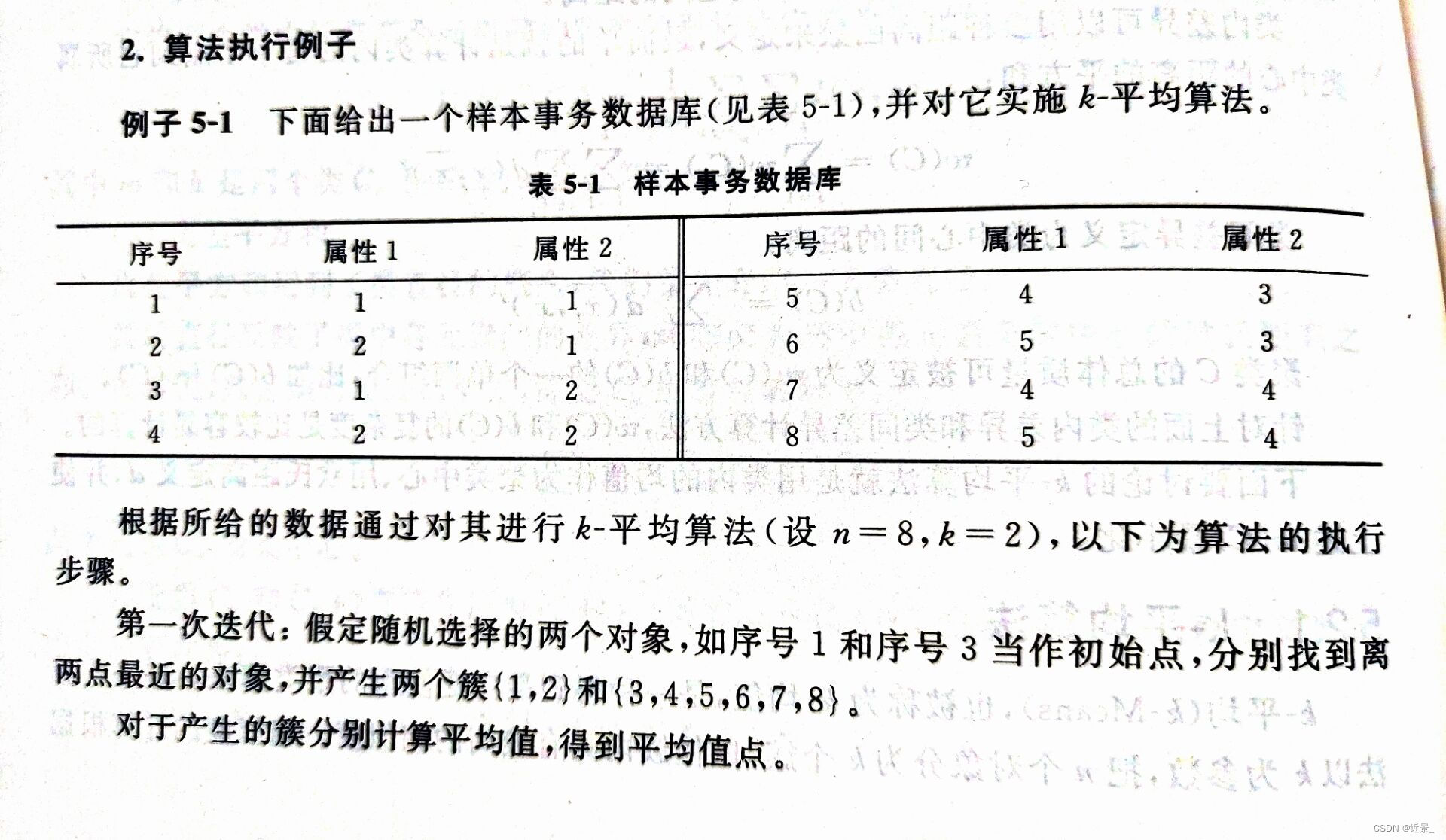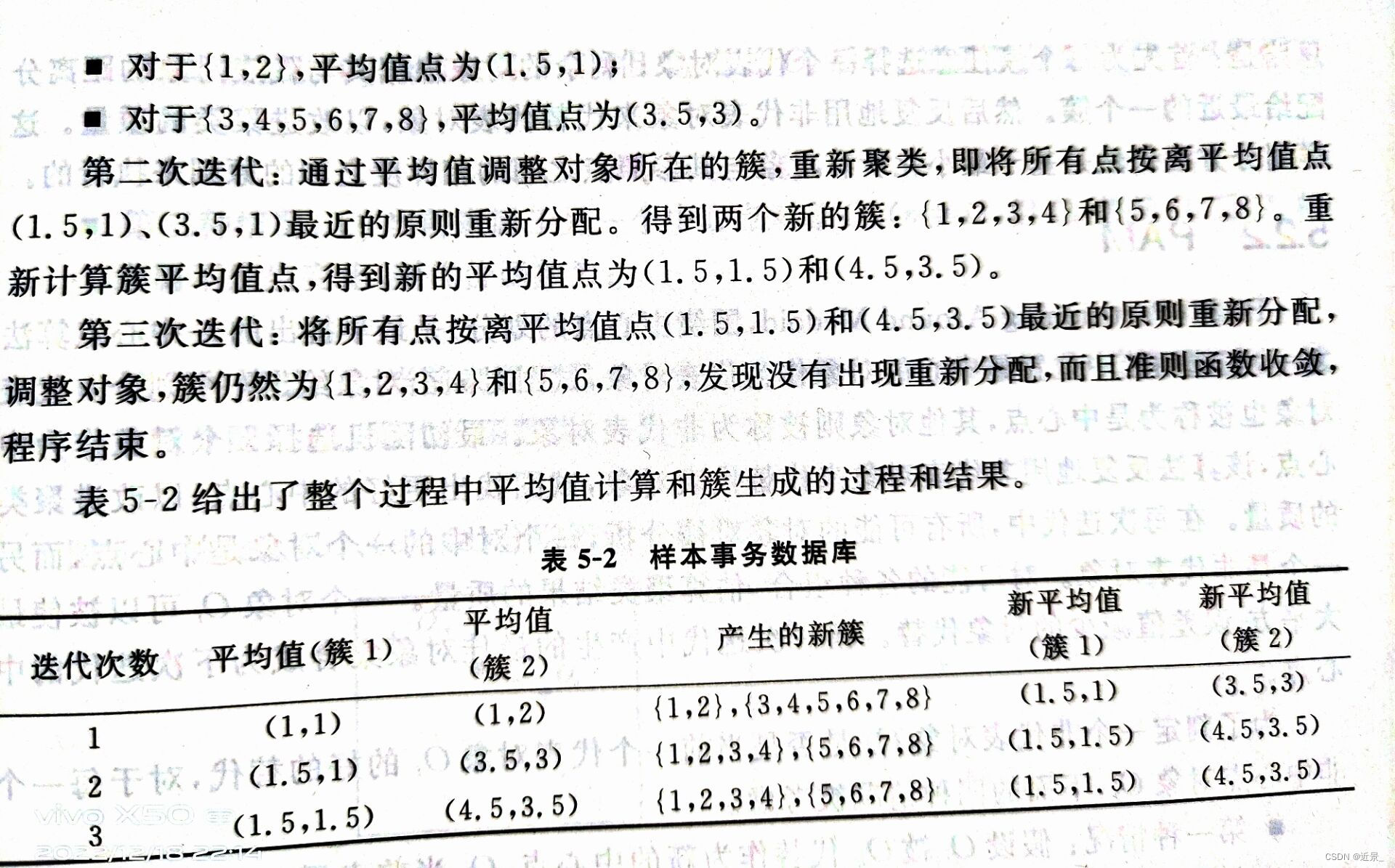## 四、K-means算法的实现过程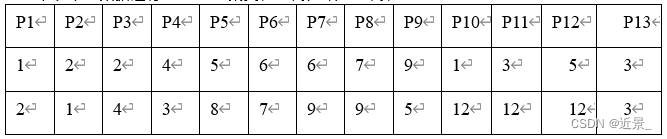（1）定义Point类，Point类中含横坐标x，纵坐标y等属性，包含静态方法getIsSame()：判断两个Point类对象是否相同、calculateDistance()方法：计算两个Point类对象之间的距离（欧氏距离）、calculateMHDDistance()方法：计算两个Point类对象之间的距离（曼哈顿距离）。定义Cluster类，在Cluster类中包含属性核心点corePoint，簇内的所有点的集合sameList。
（2）定义初始数据集dataList，定义簇的数目k，调用initDataList()方法进行初始化数据集，调用getInitCluster()方法进行初始化簇。getInitCluster()方法主要作用是获取任意k个对象作为初始簇中心，将含有k个簇的集合返回。在getInitCluster()方法体内部，定义clusterList集合用于存放k个簇，调用getRandomArray()方法获取含有k个不重复随机数的数组randomArray，数据集中k个对象的下标存放在randomArray数组中，遍历数组randomArray，取出k个任意下标的Point类对象作为相应簇cluster的核心对象点，并将每一次循环定义和实例化后的cluster添加到clusterList中，最终将clusterList集合返回。
（3）进入while循环，遍历数据集dataList中的每一项point，调用getBelongCluster()方法获取point属于的那个簇在clusterList中的下标index，取出clusterList中指定下标index的簇，将点point加入到该簇的sameList中。然后遍历数据集结束后，调用calculateClusterCore()方法计算出新的簇中心并判断出簇集合中每个簇的点集合是否有发生变化，若未发生变化，则跳出while循环，表明K-means聚类结束，反之则进入下次while循环，在遍历数据集之前，要将clusterList集合中的每一项cluster的sameList集合清空。
（4）遍历clusetrList集合，将集合中的每一项cluster输出即可。
（5）getBelongCluster()方法主要作用是获取某个点属于哪个簇的下标。在方法体内部，定义了变量closestDistance和变量resultClusterIndex分别用于存放point距离簇中心最近的距离，以及point属于的哪个簇的下标。遍历簇集合clusterList，调用Point类内静态方法calculateDistance()计算点point距离簇cluster核心点的距离赋值给distance，将第一次遍历得到的distance值赋值给cloestDistance，后面的遍历如果distance小于closestDistance，就将distance赋值给closestDistance，同时将index赋值给resultClusterIndex，循环遍历结束，最终将resultClusterIndex返回。
（6）calculateClusterCore()方法主要作用是计算出新的簇中心并返回簇的点集合是否有变化。在方法体内部定义标志变量flag，然后遍历clusterList集合中的每一项cluster，定义变量sumX和变量sumY分别用于存放簇中点集合所有的x坐标之和，以及簇中点集合所有的y坐标之和，对sumX和sumY求均值后赋值给新的簇中心点clusterCore，调用Point类内静态方法getIsSame()判断clusterCore和原簇中心是否相同，若不相同则将flag赋值为true。当遍历簇集合循环结束后，将flag值返回。要注意的是这里形参类型是List集合，传的是List集合的地址，在方法体内对集合进行修改则会导致实参的值也发生改变。

Cluster类

package com.data.mining.entity;

import lombok.Data;

import java.util.ArrayList;
import java.util.List;

@Data
public class Cluster {
private Point corePoint;
private List<Point> sameList = new ArrayList<>();

public Cluster(){}

public Cluster(Point cp){
corePoint = cp;
}
}


Point类

package com.data.mining.entity;

import lombok.Data;

@Data
public class Point {
private double x;
private double y;

public Point(){}

public Point(double x, double y){
this.x = x;
this.y = y;
}

public static boolean getIsSame(Point p1, Point p2){
if (p1.getX() == p2.getX() && p1.getY() == p2.getY()) return true;
return false;
}

public static double calculateDistance(Point p1, Point p2){
double xDistance = p1.getX() - p2.getX();
double yDistance = p1.getY() - p2.getY();
double tmp = xDistance * xDistance + yDistance * yDistance;
return Math.sqrt(tmp);
}

public static double calculateMHDDistance(Point p1, Point p2){
return Math.abs(p1.getX() - p2.getX()) + Math.abs(p1.getY() - p2.getY());
}

}


K-means算法实现代码

package com.data.mining.main;

import com.data.mining.entity.Cluster;
import com.data.mining.entity.Point;

import java.util.ArrayList;
import java.util.Arrays;
import java.util.List;
import java.util.Random;

public class Kmeans {
//定义初始数据集
public static List<Point> dataList = new ArrayList<>();
//定义簇的数目
public static Integer k = 3;

public static void main(String[] args) {
//初始化数据集和初始簇
initDataList();
List<Cluster> clusterList = getInitCluster();
while(true){
for (int j = 0; j < k; j++) {
clusterList.get(j).getSameList().clear();
}
for (Point point : dataList) {
int index = getBelongCluster(point, clusterList); //获取point属于的那个簇在clusterList中的下标
}
if (!calculateClusterCore(clusterList)) break;
}
for (Cluster cluster : clusterList) {
System.out.println(cluster);
}
}

/**
* 计算出新的簇中心并返回簇的点集合是否有变化
* @param clusterList
* @return
*/
public static boolean calculateClusterCore(List<Cluster> clusterList){
boolean flag = false;
//遍历簇集合中的每一项，更新其簇中心
for (Cluster cluster : clusterList) {
List<Point> sameList = cluster.getSameList();
double sumX = 0; //存放簇中点集合所有的X坐标之和
double sumY = 0; //存放簇中点集合所有的Y坐标之和
for (Point point : sameList) {
sumX += point.getX();
sumY += point.getY();
}
//更新簇的中心
Point clusterCore = new Point(sumX * 1.0 / sameList.size(), sumY * 1.0 / sameList.size());
if (!Point.getIsSame(clusterCore, cluster.getCorePoint())) flag = true;
cluster.setCorePoint(clusterCore);
}
return flag;
}

/**
* 获取某个点属于哪个簇的下标
* @param point
* @return
*/
public static int getBelongCluster(Point point, List<Cluster> clusterList){
double closestDistance = 0.0; //存放point距离簇中心最近的距离
int resultClusterIndex = 0; //存放point属于的那个簇的下标
int index = 0;
//遍历簇集合，计算point到簇中心的距离，找出point属于的簇
for (Cluster cluster : clusterList) {
double distance = Point.calculateDistance(point, cluster.getCorePoint());
if (index == 0) closestDistance = distance;
if (distance < closestDistance){
closestDistance = distance;
resultClusterIndex = index;
}
index++;
}
return resultClusterIndex;
}

/**
* 获取任意k个对象作为初始簇中心，将含有k个簇的集合返回
* @return
*/
public static List<Cluster> getInitCluster(){
List<Cluster> clusterList = new ArrayList<>();
int[] randomArray = getRandomArray();
//任意选取k个对象作为初始簇中心，数据集中k个对象的下标存放在randomArray中
for (int i = 0; i < randomArray.length; i++) {
Point point = dataList.get(randomArray[i]);
Cluster cluster = new Cluster(point);
}
return clusterList;
}

/**
* 获取含有k个不重复随机数的数组
* @return
*/
public static int[] getRandomArray(){
Random random = new Random();
int[] randomArray = new int[k];
for (int i = 0; i < k; i++) {
int randomItem = random.nextInt(12);
//为保证randomArray中存放的随机数不重复
while (Arrays.binarySearch(randomArray, randomItem) >= 0) randomItem = random.nextInt(12);
randomArray[i] = randomItem;
}
return randomArray;
}

/**
* 初始化数据集
*/
public static void initDataList(){
Point p1 = new Point(1, 2);
Point p2 = new Point(2, 1);
Point p3 = new Point(2, 4);
Point p4 = new Point(4, 3);
Point p5 = new Point(5, 8);
Point p6 = new Point(6, 7);
Point p7 = new Point(6, 9);
Point p8 = new Point(7, 9);
Point p9 = new Point(9, 5);
Point p10 = new Point(1, 12);
Point p11 = new Point(3, 12);
Point p12 = new Point(5, 12);
Point p13 = new Point(3, 3);

}
}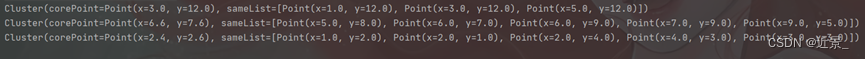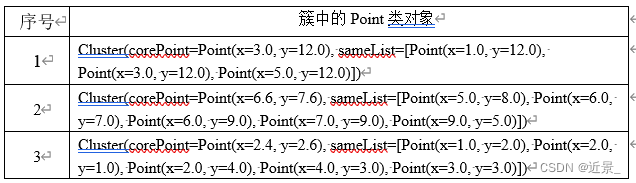## 五、实验总结

 上一篇文章      下一篇文章      查看所有文章
 加:2022-12-25 11:10:20  更:2022-12-25 11:15:40
 开发: C++知识库 Java知识库 JavaScript Python PHP知识库 人工智能 区块链 大数据 移动开发 嵌入式 开发工具 数据结构与算法 开发测试 游戏开发 网络协议 系统运维 教程: HTML教程 CSS教程 JavaScript教程 Go语言教程 JQuery教程 VUE教程 VUE3教程 Bootstrap教程 SQL数据库教程 C语言教程 C++教程 Java教程 Python教程 Python3教程 C#教程 数码: 电脑 笔记本 显卡 显示器 固态硬盘 硬盘 耳机 手机 iphone vivo oppo 小米 华为 单反 装机 图拉丁

 360图书馆 购物 三丰科技 阅读网 日历 万年历 2023年6日历 -2023/6/3 16:02:40-

 图片自动播放器 ↓图片自动播放器↓ TxT小说阅读器 ↓语音阅读,小说下载,古典文学↓ 一键清除垃圾 ↓轻轻一点,清除系统垃圾↓ 图片批量下载器 ↓批量下载图片,美女图库↓
 网站联系: qq:121756557 email:121756557@qq.com  IT数码